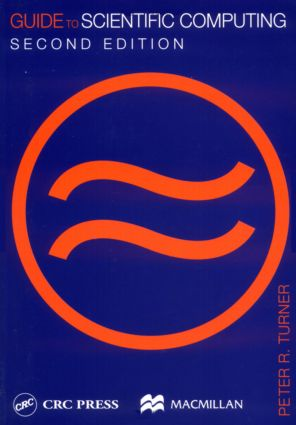# Guide To Scientific Computing

## 2nd Edition

CRC Press

312 pages

Paperback: 9780849312427
pub: 2000-12-07
SAVE ~\$14.99
\$74.95
\$59.96
x

FREE Standard Shipping!

### Description

Guide to Scientific Computing provides an introduction to the many problems of scientific computing, as well as the wide variety of methods used for their solution. It is ideal for anyone who needs an understanding of numerical mathematics or scientific computing - whether in mathematics, the sciences, engineering, or economics.

This book provides an appreciation of the need for numerical methods for solving different types of problems, and discusses basic approaches. For each of the problems mathematical justification and examples provide both practical evidence and motivations for the reader to follow. Practical justification of the methods is presented through computer examples and exercises. The major effort of programming is removed from the reader, as are the harder parts of analysis, so that the focus is clearly on the basics. Since some algebraic manipulation is unavoidable, it is carefully explained when necessary, especially in the early stages.

Guide to Scientific Computing includes an introduction to MATLAB, but the code used is not intended to exemplify sophisticated or robust pieces of software; it is purely illustrative of the methods under discussion. The book has an appendix devoted to the basics of the MATLAB package, its language and programming. The book provides an introduction to this subject which is not, in its combined demands of computing, motivation, manipulation, and analysis, paced such that only the most able can understand.

Number Representations and Errors

Introduction

Floating-Point Number

Sources of Errors

Measures of Error and Precision

Floating-Point Arithmetic

Iterative Solution of Equations

Introduction

The Bisection Method

Function Iteration

Newton's Method

The Secant Method

2 Equations in 2 Unknowns: Newton's Method

MATLAB Functions for Equation Solving

Approximate Evaluation of Functions

Introduction

Series Expansions

CORDIC Algorithms

MATLAB Functions

Interpolation

Introduction

Lagrange Interpolation

Difference Representations

Splines

MATLAB Interpolation Functions

Numerical Calculus

Introduction

Composite Formulas

Practical Numerical Integration

Improper Integrals

Numerical Differentiation

Maxima and Minima

MATLAB Functions for Numerical Calculus

Differential Equations

Introduction and Euler's Method

Runge-Kutta Methods

Multistep Methods

Systems of Differential Equations

Boundary-Value Problems (1) Shooting Methods

Boundary-Value Problems (2) Finite Difference Methods

MATLAB Functions for Ordinary Differential Equations

Linear Equations

Introduction

Gauss Elimination

LU Factorization: Iterative Refinement

Iterative Methods

Linear Least Squares Approximation

Eigenvalues

MATLAB's Linear Algebra Functions

Appendices

MATLAB Basics